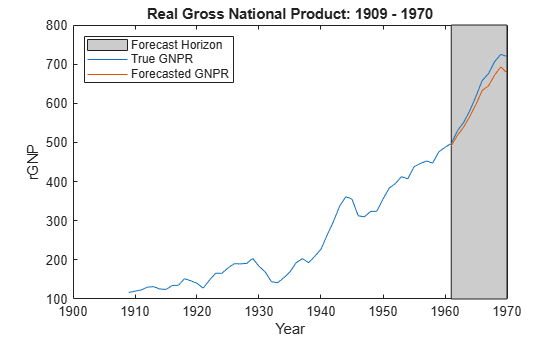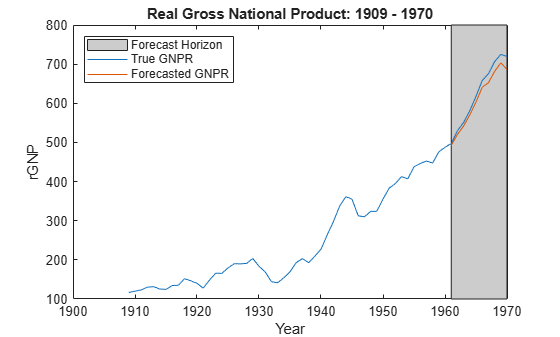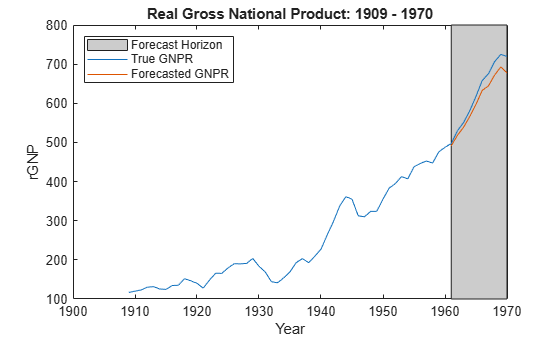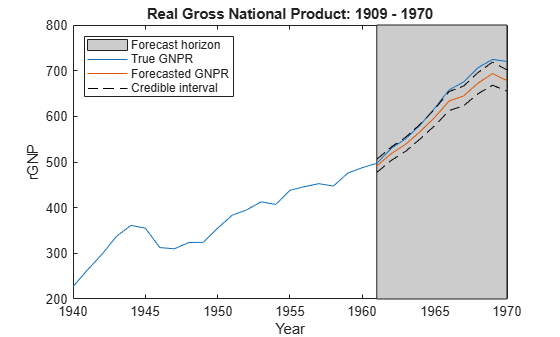# forecast

Forecast responses of Bayesian linear regression model

## Syntax

``yF = forecast(Mdl,XF)``
``yF = forecast(Mdl,XF,X,y)``
``yF = forecast(___,Name,Value)``
``````[yF,YFCov] = forecast(___)``````

## Description

example

````yF = forecast(Mdl,XF)` returns `numPeriods` forecasted responses from the Bayesian linear regression model `Mdl` given the predictor data in `XF`, a matrix with `numPeriods` rows.To estimate the forecast, `forecast` uses the mean of the `numPeriods`-dimensional posterior predictive distribution. If `Mdl` is a joint prior model (returned by `bayeslm`), then `forecast` uses only the joint prior distribution and the innovation distribution to form the predictive distribution.If `Mdl` is a posterior model (returned by `estimate`), then `forecast` uses the posterior predictive distribution. `NaN`s in the data indicate missing values, which `forecast` removes using list-wise deletion.```

example

````yF = forecast(Mdl,XF,X,y)` forecasts using the posterior predictive distribution produced or updated by incorporating the predictor data `X` and corresponding response data `y`. If `Mdl` is a joint prior model, then `forecast` produces the posterior predictive distribution by updating the prior model with information about the parameters that it obtains from the data.If `Mdl` is a posterior model, then `forecast` updates the posteriors with information about the parameters that it obtains from the additional data. The complete data likelihood is composed of the additional data `X` and `y`, and the data that created `Mdl`. ```

example

````yF = forecast(___,Name,Value)` uses any of the input argument combinations in the previous syntaxes and additional options specified by one or more name-value pair arguments. For example, you can specify a value for β or σ2 to forecast from the conditional predictive distribution of one parameter, given the specified value of the other parameter.```

example

``````[yF,YFCov] = forecast(___)``` also returns the covariance matrix of the `numPeriods`-dimensional posterior predictive distribution. Standard deviations of the forecasts are the square roots of the diagonal elements.```

## Examples

collapse all

Consider the multiple linear regression model that predicts the US real gross national product (`GNPR`) using a linear combination of industrial production index (`IPI`), total employment (`E`), and real wages (`WR`).

`${\text{GNPR}}_{t}={\beta }_{0}+{\beta }_{1}{\text{IPI}}_{t}+{\beta }_{2}{\text{E}}_{t}+{\beta }_{3}{\text{WR}}_{t}+{\epsilon }_{t}.$`

For all $t$, ${\epsilon }_{t}$ is a series of independent Gaussian disturbances with a mean of 0 and variance ${\sigma }^{2}$.

Assume these prior distributions:

• $\beta |{\sigma }^{2}\sim {N}_{4}\left(M,{\sigma }^{2}V\right)$. $M$ is a 4-by-1 vector of means, and $V$ is a scaled 4-by-4 positive definite covariance matrix.

• ${\sigma }^{2}\sim IG\left(A,B\right)$. $A$ and $B$ are the shape and scale, respectively, of an inverse gamma distribution.

These assumptions and the data likelihood imply a normal-inverse-gamma conjugate model.

Create a normal-inverse-gamma conjugate prior model for the linear regression parameters. Specify the number of predictors `p` and the variable names.

```p = 3; VarNames = ["IPI" "E" "WR"]; PriorMdl = bayeslm(p,'ModelType','conjugate','VarNames',VarNames);```

`Mdl` is a `conjugateblm` Bayesian linear regression model object representing the prior distribution of the regression coefficients and disturbance variance.

Load the Nelson-Plosser data set. Create variables for the predictor and response data. Hold out the last 10 periods of data from estimation so you can use them to forecast real GNP.

```load Data_NelsonPlosser fhs = 10; % Forecast horizon size X = DataTable{1:(end - fhs),PriorMdl.VarNames(2:end)}; y = DataTable{1:(end - fhs),'GNPR'}; XF = DataTable{(end - fhs + 1):end,PriorMdl.VarNames(2:end)}; % Future predictor data yFT = DataTable{(end - fhs + 1):end,'GNPR'}; % True future responses```

Estimate the marginal posterior distributions. Turn off the estimation display.

`PosteriorMdl = estimate(PriorMdl,X,y,'Display',false);`

`PosteriorMdl` is a `conjugateblm` model object that contains the posterior distributions of $\beta$ and ${\sigma }^{2}$.

Forecast responses by using the posterior predictive distribution and the future predictor data `XF`. Plot the true values of the response and the forecasted values.

```yF = forecast(PosteriorMdl,XF); figure; plot(dates,DataTable.GNPR); hold on plot(dates((end - fhs + 1):end),yF) h = gca; p = patch([dates(end - fhs + 1) dates(end) dates(end) dates(end - fhs + 1)],... h.YLim([1,1,2,2]),[0.8 0.8 0.8]); uistack(p,'bottom'); legend('Forecast Horizon','True GNPR','Forecasted GNPR','Location','NW') title('Real Gross National Product: 1909 - 1970'); ylabel('rGNP'); xlabel('Year'); hold off````yF` is a 10-by-1 vector of future values of real GNP corresponding to the future predictor data.

Estimate the forecast root mean squared error (RMSE).

`frmse = sqrt(mean((yF - yFT).^2))`
```frmse = 25.5397 ```

The forecast RMSE is a relative measure of forecast accuracy. Specifically, you estimate several models using different assumptions. The model with the lowest forecast RMSE is the best-performing model of the ones being compared.

Consider the regression model in Forecast Responses Using Posterior Predictive Distribution.

Create a normal-inverse-gamma semiconjugate prior model for the linear regression parameters. Specify the number of predictors `p` and the names of the regression coefficients.

```p = 3; PriorMdl = bayeslm(p,'ModelType','semiconjugate','VarNames',["IPI" "E" "WR"]);```

Load the Nelson-Plosser data set. Create variables for the response and predictor series.

```load Data_NelsonPlosser X = DataTable{:,PriorMdl.VarNames(2:end)}; y = DataTable{:,'GNPR'};```

Hold out the last 10 periods of data from estimation so you can use them to forecast real GNP. Turn off the estimation display.

```fhs = 10; % Forecast horizon size X = DataTable{1:(end - fhs),PriorMdl.VarNames(2:end)}; y = DataTable{1:(end - fhs),'GNPR'}; XF = DataTable{(end - fhs + 1):end,PriorMdl.VarNames(2:end)}; % Future predictor data yFT = DataTable{(end - fhs + 1):end,'GNPR'}; % True future responses```

Forecast responses by using the posterior predictive distribution and the future predictor data `XF`. Specify the in-sample observations `X` and `y` (the observations from which MATLAB® composes the posterior).

`yF = forecast(PriorMdl,XF,X,y)`
```yF = 10×1 491.5404 518.1725 539.0625 566.7594 597.7005 633.4666 644.7270 672.7937 693.5321 678.2268 ```

Consider the regression model in Forecast Responses Using Posterior Predictive Distribution.

Assume these prior distributions for $\mathit{k}$ = 0,...,3:

• ${\beta }_{k}|{\sigma }^{2},{\gamma }_{k}={\gamma }_{k}\sigma \sqrt{{V}_{k1}}{Z}_{1}+\left(1-{\gamma }_{k}\right)\sigma \sqrt{{V}_{k2}}{Z}_{2}$, where ${\mathit{Z}}_{1}$ and ${\mathit{Z}}_{2}$ are independent, standard normal random variables. Therefore, the coefficients have a Gaussian mixture distribution. Assume all coefficients are conditionally independent, a priori, but they are dependent on the disturbance variance.

• ${\sigma }^{2}\sim IG\left(A,B\right)$. $A$ and $B$ are the shape and scale, respectively, of an inverse gamma distribution.

• ${\gamma }_{\mathit{k}}\in \left\{0,1\right\}$and it represents the random variable-inclusion regime variable with a discrete uniform distribution.

Perform stochastic search variable selection (SSVS):

1. Create a Bayesian regression model for SSVS with a conjugate prior for the data likelihood. Use the default settings.

2. Hold out the last 10 periods of data from estimation.

3. Estimate the marginal posterior distributions.

```p = 3; PriorMdl = bayeslm(p,'ModelType','mixconjugate','VarNames',["IPI" "E" "WR"]); load Data_NelsonPlosser fhs = 10; % Forecast horizon size X = DataTable{1:(end - fhs),PriorMdl.VarNames(2:end)}; y = DataTable{1:(end - fhs),'GNPR'}; XF = DataTable{(end - fhs + 1):end,PriorMdl.VarNames(2:end)}; % Future predictor data yFT = DataTable{(end - fhs + 1):end,'GNPR'}; % True future responses rng(1); % For reproducibility PosteriorMdl = estimate(PriorMdl,X,y,'Display',false);```

Forecast responses using the posterior predictive distribution and the future predictor data `XF`. Plot the true values of the response and the forecasted values.

```yF = forecast(PosteriorMdl,XF); figure; plot(dates,DataTable.GNPR); hold on plot(dates((end - fhs + 1):end),yF) h = gca; hp = patch([dates(end - fhs + 1) dates(end) dates(end) dates(end - fhs + 1)],... h.YLim([1,1,2,2]),[0.8 0.8 0.8]); uistack(hp,'bottom'); legend('Forecast Horizon','True GNPR','Forecasted GNPR','Location','NW') title('Real Gross National Product: 1909 - 1970'); ylabel('rGNP'); xlabel('Year'); hold off````yF` is a 10-by-1 vector of future values of real GNP corresponding to the future predictor data.

Estimate the forecast root mean squared error (RMSE).

`frmse = sqrt(mean((yF - yFT).^2))`
```frmse = 18.8470 ```

The forecast RMSE is a relative measure of forecast accuracy. Specifically, you estimate several models using different assumptions. The model with the lowest forecast RMSE is the best-performing model of the ones being compared.

When you perform Bayesian regression with SSVS, a best practice is to tune the hyperparameters. One way to do so is to estimate the forecast RMSE over a grid of hyperparameter values, and choose the value that minimizes the forecast RMSE.

Consider the regression model in Forecast Responses Using Posterior Predictive Distribution.

Create a normal-inverse-gamma semiconjugate prior model for the linear regression parameters. Specify the number of predictors `p` and the names of the regression coefficients.

```p = 3; PriorMdl = bayeslm(p,'ModelType','semiconjugate','VarNames',["IPI" "E" "WR"]);```

Load the Nelson-Plosser data set. Create variables for the response and predictor series.

```load Data_NelsonPlosser X = DataTable{:,PriorMdl.VarNames(2:end)}; y = DataTable{:,'GNPR'};```

Hold out the last 10 periods of data from estimation so you can use them to forecast real GNP. Turn off the estimation display.

```fhs = 10; % Forecast horizon size X = DataTable{1:(end - fhs),PriorMdl.VarNames(2:end)}; y = DataTable{1:(end - fhs),'GNPR'}; XF = DataTable{(end - fhs + 1):end,PriorMdl.VarNames(2:end)}; % Future predictor data yFT = DataTable{(end - fhs + 1):end,'GNPR'}; % True future responses```

Forecast responses by using the conditional posterior predictive distribution of beta given ${\sigma }^{2}=2$ and using the future predictor data `XF`. Specify the in-sample observations `X` and `y` (the observations from which MATLAB® composes the posterior). Plot the true values of the response and the forecasted values.

```yF = forecast(PriorMdl,XF,X,y,'Sigma2',2); figure; plot(dates,DataTable.GNPR); hold on plot(dates((end - fhs + 1):end),yF) h = gca; hp = patch([dates(end - fhs + 1) dates(end) dates(end) dates(end - fhs + 1)],... h.YLim([1,1,2,2]),[0.8 0.8 0.8])```
```hp = Patch with properties: FaceColor: [0.8000 0.8000 0.8000] FaceAlpha: 1 EdgeColor: [0 0 0] LineStyle: '-' Faces: [1 2 3 4] Vertices: [4x2 double] Show all properties ```
```uistack(hp,'bottom'); legend('Forecast Horizon','True GNPR','Forecasted GNPR','Location','NW') title('Real Gross National Product: 1909 - 1970'); ylabel('rGNP'); xlabel('Year'); hold off```Consider the regression model in Forecast Responses Using Posterior Predictive Distribution.

Create a normal-inverse-gamma semiconjugate prior model for the linear regression parameters. Specify the number of predictors `p` and the names of the regression coefficients.

```p = 3; PriorMdl = bayeslm(p,'ModelType','semiconjugate','VarNames',["IPI" "E" "WR"]);```

Load the Nelson-Plosser data set. Create variables for the response and predictor series.

```load Data_NelsonPlosser X = DataTable{:,PriorMdl.VarNames(2:end)}; y = DataTable{:,'GNPR'};```

Hold out the last 10 periods of data from estimation so you can use them to forecast real GNP. Turn off the estimation display.

```fhs = 10; % Forecast horizon size X = DataTable{1:(end - fhs),PriorMdl.VarNames(2:end)}; y = DataTable{1:(end - fhs),'GNPR'}; XF = DataTable{(end - fhs + 1):end,PriorMdl.VarNames(2:end)}; % Future predictor data yFT = DataTable{(end - fhs + 1):end,'GNPR'}; % True future responses```

Forecast responses and their covariance matrix by using the posterior predictive distribution and the future predictor data `XF`. Specify the in-sample observations `X` and `y` (the observations from which MATLAB® composes the posterior).

`[yF,YFCov] = forecast(PriorMdl,XF,X,y);`

Because the predictive posterior distribution is not analytical, a reasonable approximation to a set of 95% credible intervals is

`${\underset{}{\overset{ˆ}{y}}}_{i}±{z}_{0.975}SE\left({\underset{}{\overset{ˆ}{y}}}_{i}\right),$`

for all $i$ in the forecast horizon. Estimate 95% credible intervals for the forecasts using this formula.

```n = sum(all(~isnan([X y]'))); cil = yF - norminv(0.975)*sqrt(diag(YFCov)); ciu = yF + norminv(0.975)*sqrt(diag(YFCov));```

Plot the data, forecasts, and forecast intervals.

```figure; plot(dates(end-30:end),DataTable.GNPR(end-30:end)); hold on h = gca; plot(dates((end - fhs + 1):end),yF) plot(dates((end - fhs + 1):end),[cil ciu],'k--') hp = patch([dates(end - fhs + 1) dates(end) dates(end) dates(end - fhs + 1)],... h.YLim([1,1,2,2]),[0.8 0.8 0.8]); uistack(hp,'bottom'); legend('Forecast horizon','True GNPR','Forecasted GNPR',... 'Credible interval','Location','NW') title('Real Gross National Product: 1909 - 1970'); ylabel('rGNP'); xlabel('Year'); hold off```## Input Arguments

collapse all

Standard Bayesian linear regression model or model for predictor variable selection, specified as a model object in this table.

Model ObjectDescription
`conjugateblm`Dependent, normal-inverse-gamma conjugate model returned by `bayeslm` or `estimate`
`semiconjugateblm`Independent, normal-inverse-gamma semiconjugate model returned by `bayeslm`
`diffuseblm`Diffuse prior model returned by `bayeslm`
`empiricalblm`Prior model characterized by samples from prior distributions, returned by `bayeslm` or `estimate`
`customblm`Prior distribution function that you declare returned by `bayeslm`
`mixconjugateblm`Dependent, Gaussian-mixture-inverse-gamma conjugate model for SSVS predictor variable selection, returned by `bayeslm`
`mixsemiconjugateblm`Independent, Gaussian-mixture-inverse-gamma semiconjugate model for SSVS predictor variable selection, returned by `bayeslm`
`lassoblm`Bayesian lasso regression model returned by `bayeslm`

Typically, model objects returned by `estimate` represent marginal posterior distributions. When you estimate a posterior by using `estimate`, if you specify estimation of a conditional posterior, then `estimate` returns the prior model.

Forecast-horizon predictor data, specified as a `numPeriods`-by-`PriorMdl.NumPredictors` numeric matrix. `numPeriods` determines the length of the forecast horizon. The columns of `XF` correspond to the columns of any other predictor data set, that is, `X` or data used to form the posterior distribution in `Mdl`.

Data Types: `double`

Predictor data for the multiple linear regression model, specified as a `numObservations`-by-`PriorMdl.NumPredictors` numeric matrix. `numObservations` is the number of observations and must be equal to the length of `y`.

If `Mdl` is a posterior distribution, then the columns of `X` must correspond to the columns of the predictor data used to estimate the posterior.

Data Types: `double`

Response data for the multiple linear regression model, specified as a numeric vector with `numObservations` elements.

Data Types: `double`

### Name-Value Arguments

Specify optional pairs of arguments as `Name1=Value1,...,NameN=ValueN`, where `Name` is the argument name and `Value` is the corresponding value. Name-value arguments must appear after other arguments, but the order of the pairs does not matter.

Before R2021a, use commas to separate each name and value, and enclose `Name` in quotes.

Example: `'Sigma2',2` specifies forecasting from the conditional predictive distribution given the specified disturbance variance of `2`.

Options for All Models Except For Empirical

collapse all

Value of the regression coefficients for forecasting from the conditional predictive distribution given the regression coefficients, specified as the comma-separated pair consisting of `'Beta'` and an (`Mdl.Intercept` + `Mdl.NumPredictors`)-by-1 numeric vector. When using a posterior distribution, `forecast` forecasts from $\pi \left(\stackrel{^}{y}|y,X,\beta =Beta\right)$, where `y` is `y`, `X` is `X`, and `Beta` is the value of `'Beta'`. If `Mdl.Intercept` is `true`, then `Beta(1)` corresponds to the model intercept. All other values correspond to the predictor variables that compose the columns of `X`.

You cannot specify `Beta` and `Sigma2` simultaneously.

By default, `forecast` does not forecast from the conditional predictive distribution given β.

Example: `'Beta',1:3`

Data Types: `double`

Value of the disturbance variance forecasting from the conditional predictive distribution given the disturbance variance, specified as the comma-separated pair consisting of `'Sigma2'` and a positive numeric scalar. When using a posterior distribution, `forecast` draws from $\pi \left(\stackrel{^}{y}|y,X,{\sigma }^{2}=Sigma2\right)$, where `y` is `y`, `X` is `X`, and `Sigma2` is the value of `'Sigma2'`.

You cannot specify `Sigma2` and `Beta` simultaneously.

By default, `forecast` does not draw from the conditional predictive posterior of σ2.

Example: `'Sigma2',1`

Data Types: `double`

Options for All Models Except Conjugate

collapse all

Monte Carlo simulation adjusted sample size, specified as the comma-separated pair consisting of `'NumDraws'` and a positive integer. `forecast` actually draws `BurnIn``NumDraws*``Thin` samples. Therefore, `forecast` bases the estimates off `NumDraws` samples. For details on how `forecast` reduces the full Monte Carlo sample, see Algorithms.

If `Mdl` is a `semiconjugateblm` model and you specify `Beta` or `Sigma2`, then MATLAB® ignores `NumDraws`.

Example: `'NumDraws',1e7`

Data Types: `double`

Options for All Models Except Conjugate and Empirical

collapse all

Number of draws to remove from the beginning of the Monte Carlo sample to reduce transient effects, specified as the comma-separated pair consisting of `'BurnIn'` and a nonnegative scalar. For details on how `forecast` reduces the full Monte Carlo sample, see Algorithms.

Tip

1. Determine the extent of the transient behavior in the sample by specifying `'BurnIn',0`.

2. Simulate a few thousand observations by using `forecast`.

3. Draw trace plots.

Example: `'BurnIn',0`

Data Types: `double`

Monte Carlo adjusted sample size multiplier, specified as the comma-separated pair consisting of `'Thin'` and a positive integer.

The actual Monte Carlo sample size is `BurnIn` + `NumDraws``*Thin`. After discarding the burn-in, `forecast` discards every `Thin``1` draws, and then retains the next draw. For details on how `forecast` reduces the full Monte Carlo sample, see Algorithms.

Tip

To reduce potential large serial correlation in the Monte Carlo sample, or to reduce the memory consumption of the draws stored in `Mdl`, specify a large value for `Thin`.

Example: `'Thin',5`

Data Types: `double`

Starting values of the regression coefficients for the Markov chain Monte Carlo (MCMC) sample, specified as the comma-separated pair consisting of `'BetaStart'` and a numeric column vector with (`PriorMdl.Intercept` + `PriorMdl.NumPredictors`) elements. By default, `BetaStart` is the ordinary least-squares (OLS) estimate.

Tip

A good practice is to run `forecast` multiple times using different parameter starting values. Verify that the solutions from each run converge to similar values.

Example: `'BetaStart',[1; 2; 3]`

Data Types: `double`

Starting values of the disturbance variance for the MCMC sample, specified as the comma-separated pair consisting of `'Sigma2Start'` and a positive numeric scalar. By default, `Sigma2Start` is the OLS residual mean squared error.

Tip

A good practice is to run `forecast` multiple times using different parameter starting values. Verify that the solutions from each run converge to similar values.

Example: `'Sigma2Start',4`

Data Types: `double`

Options for Custom Models

collapse all

Reparameterization of σ2 as log(σ2) during posterior estimation and simulation, specified as the comma-separated pair consisting of `'Reparameterize'` and a value in this table.

ValueDescription
`false``forecast` does not reparameterize σ2.
`true``forecast` reparameterizes σ2 as log(σ2). `forecast` converts results back to the original scale and does not change the functional form of `PriorMdl.LogPDF`.

Tip

If you experience numeric instabilities during the posterior estimation or simulation of σ2, then specify `'Reparameterize',true`.

Example: `'Reparameterize',true`

Data Types: `logical`

MCMC sampler, specified as the comma-separated pair consisting of `'Sampler'` and a value in this table.

ValueDescription
`'slice'`Slice sampler
`'metropolis'`Random walk Metropolis sampler
`'hmc'`Hamiltonian Monte Carlo (HMC) sampler

Tip

• To increase the quality of the MCMC draws, tune the sampler.

1. Before calling `forecast`, specify the tuning parameters and their values by using `sampleroptions`. For example, to specify the slice sampler width `width`, use:

`options = sampleroptions('Sampler',"slice",'Width',width);`

2. Specify the object containing the tuning parameter specifications returned by `sampleroptions` by using the `'Options'` name-value pair argument. For example, to use the tuning parameter specifications in `options`, specify:

``'Options',options``

• If you specify the HMC sampler, then a best practice is to provide the gradient for some variables, at least. `forecast` resorts the numerical computation of any missing partial derivatives (`NaN` values) in the gradient vector.

Example: `'Sampler',"hmc"`

Data Types: `string`

Sampler options, specified as the comma-separated pair consisting of `'Options'` and a structure array returned by `sampleroptions`. Use `'Options'` to specify the MCMC sampler and its tuning-parameter values.

Example: `'Options',sampleroptions('Sampler',"hmc")`

Data Types: `struct`

Typical sampling-interval width around the current value in the marginal distributions for the slice sampler, specified as the comma-separated pair consisting of `'Width'` and a positive numeric scalar or a (`PriorMdl.Intercept` + `PriorMdl.NumPredictors` + `1`)-by-1 numeric vector of positive values. The first element corresponds to the model intercept, if one exists in the model. The next `PriorMdl.NumPredictors` elements correspond to the coefficients of the predictor variables ordered by the predictor data columns. The last element corresponds to the model variance.

• If `Width` is a scalar, then `forecast` applies `Width` to all `PriorMdl.NumPredictors` + `PriorMdl.Intercept` + `1` marginal distributions.

• If `Width` is a numeric vector, then `forecast` applies the first element to the intercept (if one exists), the next `PriorMdl.NumPredictors` elements to the regression coefficients corresponding to the predictor variables in `X`, and the last element to the disturbance variance.

• If the sample size (`size(X,1)`) is less than 100, then `Width` is `10` by default.

• If the sample size is at least 100, then `forecast` sets `Width` to the vector of corresponding posterior standard deviations by default, assuming a diffuse prior model (`diffuseblm`).

The typical width of the slice sampler does not affect convergence of the MCMC sample. It does affect the number of required function evaluations, that is, the efficiency of the algorithm. If the width is too small, then the algorithm can implement an excessive number of function evaluations to determine the appropriate sampling width. If the width is too large, then the algorithm might have to decrease the width to an appropriate size, which requires function evaluations.

`forecast` sends `Width` to the `slicesample` function. For more details, see `slicesample`.

Tip

• For maximum flexibility, specify the slice sampler width `width` by using the `'Options'` name-value pair argument. For example:

`'Options',sampleroptions('Sampler',"slice",'Width',width)`

Example: `'Width',[100*ones(3,1);10]`

## Output Arguments

collapse all

Forecasted responses (the mean of the predictive distribution), returned as a `numPeriods`-by-1 numeric vector. Rows correspond to the rows of `XF`.

Covariance matrix of the predictive distribution, returned as a `numPeriods`-by-`numPeriods` numeric, symmetric, positive definite matrix. Rows and columns correspond to the rows of `yF`.

To obtain a vector of standard deviations for the forecasted responses, enter `sqrt(diag(YFCov))`.

## Limitations

If `Mdl` is an `empiricalblm` model object, then you cannot specify `Beta` or `Sigma2`. You cannot forecast from conditional predictive distributions by using an empirical prior distribution.

collapse all

### Bayesian Linear Regression Model

A Bayesian linear regression model treats the parameters β and σ2 in the multiple linear regression (MLR) model yt = xtβ + εt as random variables.

For times t = 1,...,T:

• yt is the observed response.

• xt is a 1-by-(p + 1) row vector of observed values of p predictors. To accommodate a model intercept, x1t = 1 for all t.

• β is a (p + 1)-by-1 column vector of regression coefficients corresponding to the variables that compose the columns of xt.

• εt is the random disturbance with a mean of zero and Cov(ε) = σ2IT×T, while ε is a T-by-1 vector containing all disturbances. These assumptions imply that the data likelihood is

`$\ell \left(\beta ,{\sigma }^{2}|y,x\right)=\prod _{t=1}^{T}\varphi \left({y}_{t};{x}_{t}\beta ,{\sigma }^{2}\right).$`

ϕ(yt;xtβ,σ2) is the Gaussian probability density with mean xtβ and variance σ2 evaluated at yt;.

Before considering the data, you impose a joint prior distribution assumption on (β,σ2). In a Bayesian analysis, you update the distribution of the parameters by using information about the parameters obtained from the likelihood of the data. The result is the joint posterior distribution of (β,σ2) or the conditional posterior distributions of the parameters.

## Tips

• Monte Carlo simulation is subject to variation. If `forecast` uses Monte Carlo simulation, then estimates and inferences might vary when you call `forecast` multiple times under seemingly equivalent conditions. To reproduce estimation results, set a random number seed by using `rng` before calling `forecast`.

• If `forecast` issues an error while estimating the posterior distribution using a custom prior model, then try adjusting initial parameter values by using `BetaStart` or `Sigma2Start`, or try adjusting the declared log prior function, and then reconstructing the model. The error might indicate that the log of the prior distribution is `–Inf` at the specified initial values.

• To forecasted responses from the conditional posterior predictive distribution of analytically intractable models, except empirical models, pass your prior model object and the estimation-sample data to `forecast`. Then, specify the `Beta` name-value pair argument to forecast from the conditional posterior of σ2, or specify the `Sigma2` name-value pair argument to forecast from the conditional posterior of β.

## Algorithms

• Whenever `forecast` must estimate a posterior distribution (for example, when `Mdl` represents a prior distribution and you supply `X` and `y`) and the posterior is analytically tractable, `forecast` evaluates the closed-form solutions to Bayes estimators. Otherwise, `forecast` resorts to Monte Carlo simulation to forecast by using the posterior predictive distribution. For more details, see Posterior Estimation and Inference.

• This figure illustrates how `forecast` reduces the Monte Carlo sample using the values of `NumDraws`, `Thin`, and `BurnIn`. Rectangles represent successive draws from the distribution. `forecast` removes the white rectangles from the Monte Carlo sample. The remaining `NumDraws` black rectangles compose the Monte Carlo sample.## Version History

Introduced in R2017a